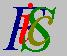Mauro Murzi's pages on Philosophy of Science - Quantum mechanics
State description in classical mechanicsPartial derivatives Quantum operators# 3. Schrödinger equations.

A brief presentation of the state description in classical physics precedes the analysis of the rules that associate a quantum operator to a classical physical quantity; then the first Schrödinger equation is introduced and, as an example of application of the equation, the formula for Bohr energy levels is derived. The section ends with an introduction to the state description in quantum mechanics and the role of the second Schrödinger equation.

## State description in classical mechanics.

Consider a classical particle (that is a particle moving according to Newton's laws of motion), with a given mass m, that is subjected to a field of force. Suppose that:

• the position and the momentum of that particle at a given time t0 are known
• the mathematical expression of the field acting on the particle is known.

Then, according to classical physics, it is possible to determine:

• the position and the momentum of the particle at every time t
• every other mechanical quantity at every time t.

If the initial position and momentum of a particle are known, with the exact expression of the forces acting on that particle, then the position and the momentum of the particle can be calculated at every time in the future and in the past. Since every classical mechanical quantity is uniquely defined by means of position and momentum, which are known at every time, then also the value of every classical mechanical quantity at every time is known.

In classical physics, the position of a particle with respect to a given frame of reference is expressed by means of a vector q or by means of the three components q1 , q2 , q3 of this vector.

The momentum of a particle is expressed by the vector p=mv, where v is the velocity of the particle with respect to the given frame of reference. The three components of the vector p are denoted p1 , p2 , p3 .

The mechanical energy E of the particle is given by the sum of kinetic energy T and potential energy U. The following relations hold:

• (3.1)  E = T + U
• (3.2)  T = ½ m v² = ½ p²/m = (p1² + p2² + p3²) / (2m)
• (3.3)  E = (p1² + p2² + p3²) / (2m) + U

Relations (3.1)-(3.3), derived in a simple classical system, are nevertheless useful in quantum mechanics.Partial derivatives Quantum operators